# MCAT Physical : Harmonics

## Example Questions

### Example Question #1 : Harmonics

A string of length 2.5m is struck producing a harmonic. The wavelength produced is 1.25m. Which harmonic is the string vibrating at?

First

Zeroth

Fourth

Second

Fourth

Explanation:

A harmonic is a standing wave that has certain points (nodes) which do not go through any displacement, and certain points (antinodes) that are moving through maximum displacement during the wave's resonation.

Wavelength is related to harmonic on a string fixed at both ends through the formula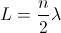, whereis the length of the string andis the number harmonic. By plugging in the given length and wavelength, we can solve for.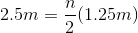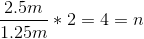The string must be vibrating at the fourth harmonic.

### Example Question #1 : Harmonics

At a local concert, a speaker is set up to produce low-pitched base sounds with a frequency range of 20Hz to 200Hz, which can be modeled as  sine waves. In a simplified model, the sound waves the speaker produces can be modeled as a cylindrical pipe with one end closed that travel through the air at a velocity of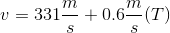, where T is the temperature in °C.

How are the first three harmonics of the base speaker designated?

1, 3, 5

1, 2, 5

1, 2, 3

1, 3, 4

1, 3, 5

Explanation:

First, notice that the paragraph above tells us that the wave can be modeled as a pipe with one end closed. This is in contrast to the other possibility, where the wave is modeled as a pipe with two ends open. It is critical to recognize this difference, as the definition of sequential harmonics and the formula used to calculate them changes depending on whether both ends are open or not. In the situation where one end is closed, the harmonics are odd numbers, meaning that the first three harmonics are 1st, 3rd, and 5th. In the situation where both ends are open or both ends are closed, the harmonics are sequential, meaning that the first three harmonics are 1st, 2nd, and 3rd.

### Example Question #1 : Harmonics

At a local concert, a speaker is set up to produce low-pitched base sounds with a frequency range of 20Hz to 200Hz, which can be modeled as  sine waves. In a simplified model, the sound waves the speaker produces can be modeled as a cylindrical pipe with one end closed that travel through the air at a velocity of, where T is the temperature in °C.

What is the closest distance a person could be standing away from the speaker in order to hear the loudest fundamental frequency of a 1.2m wavelength wave at the concert?

1.1m

1.9m

0.3m

0.7m

0.3m

Explanation:

This question asks us to incorporate information we learned in the pre-question text and new information in the question. First, we are told that we are looking to calculate a distance where maximal sound is heard, in other words, where the amplitude is at its maximum. This only occurs at an anti-node.

Additionally, we know that the speaker can be modeled as a one-end closed pipe, meaning that the wavelengths of the harmonics are calculated as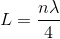.

In a one-end closed pipe model, each harmonic ends with maximal amplitude, so we can find L (the length of the pipe, i.e. where the person standing would hear maximal sound), as we know the fundamental harmonic has n = 1.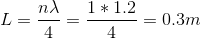### Example Question #1 : Harmonics

If the first overtone of a sound wave in a pipe with one end open and one end closed has a frequency of 300Hz, what is the frequency of the second overtone?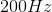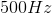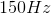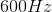In an open-closed pipe, we get only odd harmonics, since there must always be a displacement node at the closed end and a displacement antinode at the open end. So, the first overtone is the same as the third harmonic, which has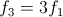. Similarly, the second overtone is the fifth harmonic, which has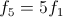. Using the given value of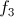in the first overtone to be 300Hz, we find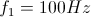and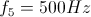.